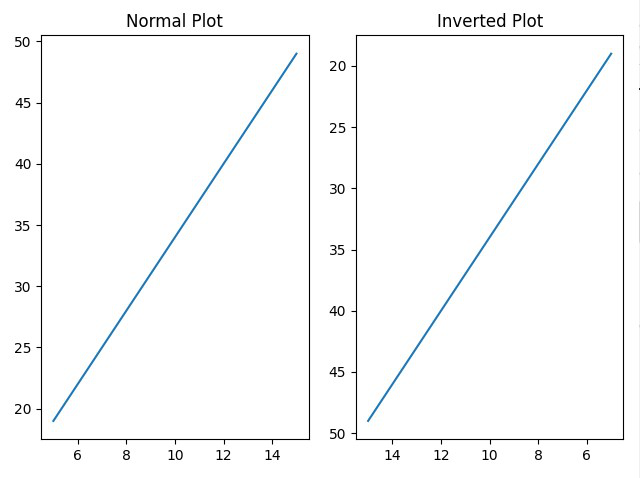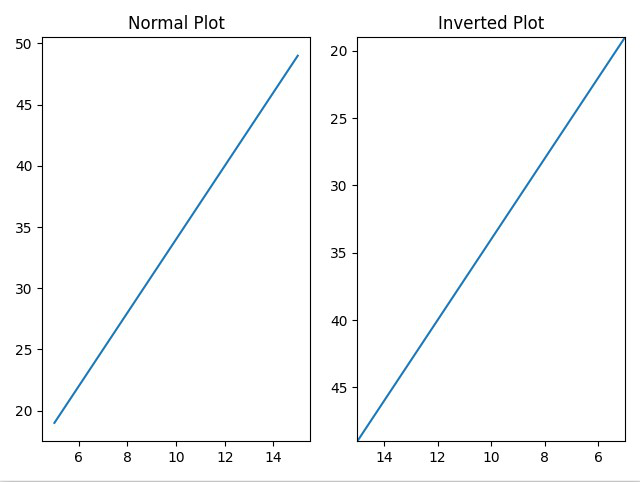Related Articles

# How to Reverse Axes in Matplotlib?

• Difficulty Level : Easy
• Last Updated : 03 Jan, 2021

In Matplotlib we can reverse axes of a graph using multiple methods. Most common method is by using invert_xaxis() and invert_yaxis() for the axes objects.  Other than that we can also use xlim() and ylim(), and axis() methods for the pyplot object.

Method 1: Using invert_xaxis() and invert_yaxis() method

To invert X-axis and Y-axis, we can use invert_xaxis() and invert_yaxis() function. We can invert either any one of the axes or both axes using the above methods.

Code:

## Python3

 `# importing numpy and matplotlib``import` `numpy as np``import` `matplotlib.pyplot as plt`` ` `# creating an x sequence``x ``=` `np.linspace(``5``, ``15``, ``35``)`` ` `# equation of a straigt line``y ``=` `3``*``x``+``4`` ` `# creating graph space for two graphs``graph, (plot1, plot2) ``=` `plt.subplots(``1``, ``2``)`` ` `# plot1 graph for normal axes``plot1.plot(x, y)``plot1.set_title(``"Normal Plot"``)`` ` `# plot2 graph for inverted axes``plot2.plot(x, y)``plot2.set_title(``"Inverted Plot"``)``plot2.invert_xaxis()``plot2.invert_yaxis()`` ` `# display the graph``graph.tight_layout()``plt.show()`

Output:Normal Plot and Inverted Plot (Both axes inverted)

Method 2: Using xlim() and ylim() method

xlim() and ylim() can also be used to invert axes of a graph plot. They are generally used to set or get limits for X-axis and Y-axis respectively. But if we pass the minimum value in the axis as the upper limit and maximum value in the axis as the lower limit we can get a reverted axis.

Code:

## Python3

 `# importing numpy and matplotlib``import` `numpy as np``import` `matplotlib.pyplot as plt`` ` `# creating an x sequence``x ``=` `np.linspace(``5``, ``15``, ``35``)`` ` `# equation of a straigt line``y ``=` `3``*``x``+``4`` ` `# creating graph space for two graphs``graph, (plot1, plot2) ``=` `plt.subplots(``1``, ``2``)`` ` `# plot1 graph for normal axes``plot1.plot(x, y)``plot1.set_title(``"Normal Plot"``)`` ` `# plot2 graph for inverted axes``plot2.plot(x, y)``plot2.set_title(``"Inverted Plot"``)``plt.xlim(``max``(x), ``min``(x))``plt.ylim(``max``(y), ``min``(y))`` ` `# display the graph``graph.tight_layout()``plt.show()`

Output:Normal Plot and Inverted Plot (Both axes inverted)

Method 3: Using axis() method

Similar to xlim() and ylim(), axis() method is also used to set the minimum and maximum values of the X-axis and Y-axis. So, if we pass the minimum value in the axis as the upper limit and the maximum value in the axis as the lower limit we can get a reverted axis.

Code:

## Python3

 `# importing numpy and matplotlib``import` `numpy as np``import` `matplotlib.pyplot as plt`` ` `# creating an x sequence``x ``=` `np.linspace(``5``, ``15``, ``35``)`` ` `# equation of a straigt line``y ``=` `3``*``x``+``4`` ` `# creating graph space for two graphs``graph, (plot1, plot2) ``=` `plt.subplots(``1``, ``2``)`` ` `# plot1 graph for normal axes``plot1.plot(x, y)``plot1.set_title(``"Normal Plot"``)`` ` `# plot2 graph for inverted axes``plot2.plot(x, y)``plot2.set_title(``"Inverted Plot"``)``plt.axis([``max``(x), ``min``(x), ``max``(y), ``min``(y)])`` ` `# display the graph``graph.tight_layout()``plt.show()`

Output:Attention geek! Strengthen your foundations with the Python Programming Foundation Course and learn the basics.

To begin with, your interview preparations Enhance your Data Structures concepts with the Python DS Course. And to begin with your Machine Learning Journey, join the Machine Learning – Basic Level Course

My Personal Notes arrow_drop_up